Anúncio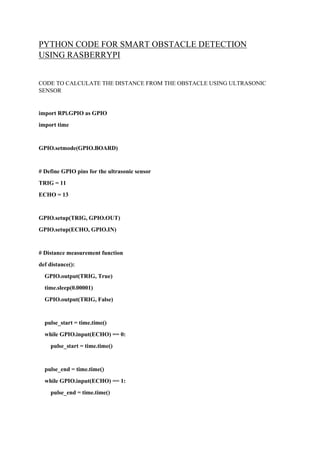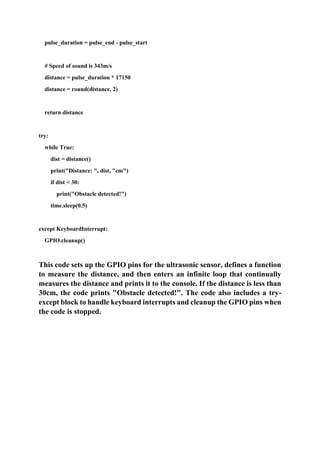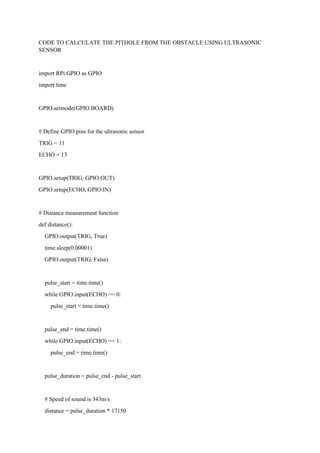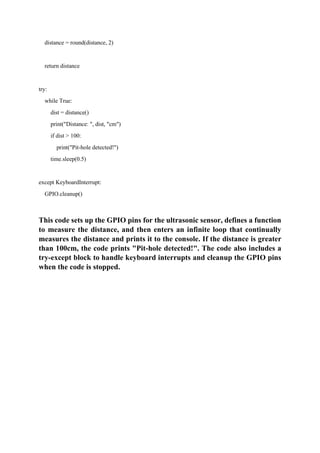Anúncio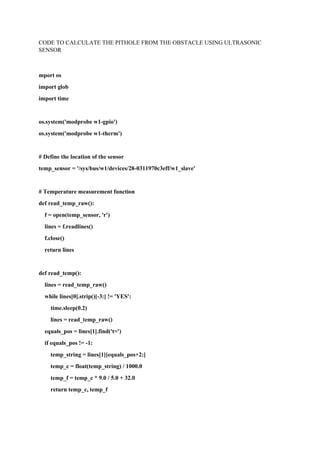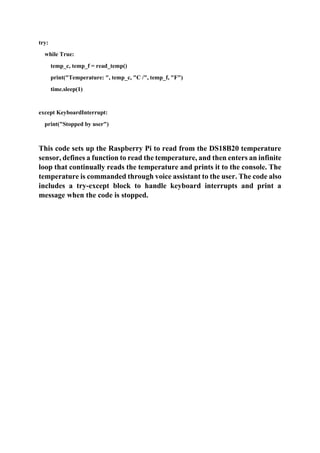Próximos SlideShares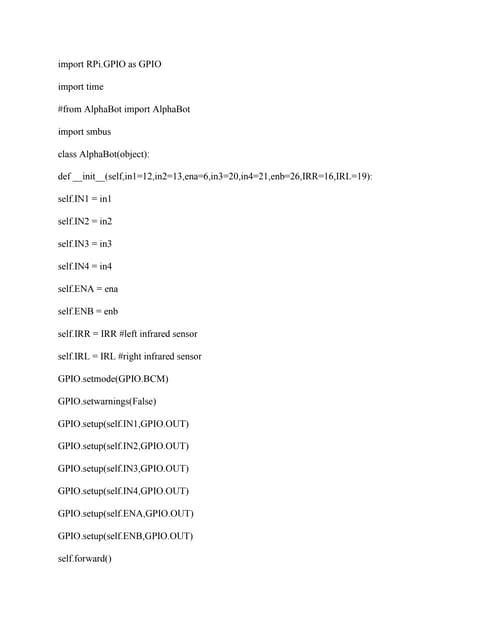import RPi-GPIO as GPIO import time #from AlphaBot import AlphaBot imp.pdf
Carregando em ... 3
1 de 6
Anúncio

### PYTHON CODE FOR SMART OBSTACLE DETECTION USING RASBERRYPI.pdf

1. PYTHON CODE FOR SMART OBSTACLE DETECTION USING RASBERRYPI CODE TO CALCULATE THE DISTANCE FROM THE OBSTACLE USING ULTRASONIC SENSOR import RPi.GPIO as GPIO import time GPIO.setmode(GPIO.BOARD) # Define GPIO pins for the ultrasonic sensor TRIG = 11 ECHO = 13 GPIO.setup(TRIG, GPIO.OUT) GPIO.setup(ECHO, GPIO.IN) # Distance measurement function def distance(): GPIO.output(TRIG, True) time.sleep(0.00001) GPIO.output(TRIG, False) pulse_start = time.time() while GPIO.input(ECHO) == 0: pulse_start = time.time() pulse_end = time.time() while GPIO.input(ECHO) == 1: pulse_end = time.time()
2. pulse_duration = pulse_end - pulse_start # Speed of sound is 343m/s distance = pulse_duration * 17150 distance = round(distance, 2) return distance try: while True: dist = distance() print("Distance: ", dist, "cm") if dist < 30: print("Obstacle detected!") time.sleep(0.5) except KeyboardInterrupt: GPIO.cleanup() This code sets up the GPIO pins for the ultrasonic sensor, defines a function to measure the distance, and then enters an infinite loop that continually measures the distance and prints it to the console. If the distance is less than 30cm, the code prints "Obstacle detected!". The code also includes a try- except block to handle keyboard interrupts and cleanup the GPIO pins when the code is stopped.
3. CODE TO CALCULATE THE PITHOLE FROM THE OBSTACLE USING ULTRASONIC SENSOR import RPi.GPIO as GPIO import time GPIO.setmode(GPIO.BOARD) # Define GPIO pins for the ultrasonic sensor TRIG = 11 ECHO = 13 GPIO.setup(TRIG, GPIO.OUT) GPIO.setup(ECHO, GPIO.IN) # Distance measurement function def distance(): GPIO.output(TRIG, True) time.sleep(0.00001) GPIO.output(TRIG, False) pulse_start = time.time() while GPIO.input(ECHO) == 0: pulse_start = time.time() pulse_end = time.time() while GPIO.input(ECHO) == 1: pulse_end = time.time() pulse_duration = pulse_end - pulse_start # Speed of sound is 343m/s distance = pulse_duration * 17150
4. distance = round(distance, 2) return distance try: while True: dist = distance() print("Distance: ", dist, "cm") if dist > 100: print("Pit-hole detected!") time.sleep(0.5) except KeyboardInterrupt: GPIO.cleanup() This code sets up the GPIO pins for the ultrasonic sensor, defines a function to measure the distance, and then enters an infinite loop that continually measures the distance and prints it to the console. If the distance is greater than 100cm, the code prints "Pit-hole detected!". The code also includes a try-except block to handle keyboard interrupts and cleanup the GPIO pins when the code is stopped.
5. CODE TO CALCULATE THE PITHOLE FROM THE OBSTACLE USING ULTRASONIC SENSOR mport os import glob import time os.system('modprobe w1-gpio') os.system('modprobe w1-therm') # Define the location of the sensor temp_sensor = '/sys/bus/w1/devices/28-0311970c3eff/w1_slave' # Temperature measurement function def read_temp_raw(): f = open(temp_sensor, 'r') lines = f.readlines() f.close() return lines def read_temp(): lines = read_temp_raw() while lines.strip()[-3:] != 'YES': time.sleep(0.2) lines = read_temp_raw() equals_pos = lines.find('t=') if equals_pos != -1: temp_string = lines[equals_pos+2:] temp_c = float(temp_string) / 1000.0 temp_f = temp_c * 9.0 / 5.0 + 32.0 return temp_c, temp_f
6. try: while True: temp_c, temp_f = read_temp() print("Temperature: ", temp_c, "C /", temp_f, "F") time.sleep(1) except KeyboardInterrupt: print("Stopped by user") This code sets up the Raspberry Pi to read from the DS18B20 temperature sensor, defines a function to read the temperature, and then enters an infinite loop that continually reads the temperature and prints it to the console. The temperature is commanded through voice assistant to the user. The code also includes a try-except block to handle keyboard interrupts and print a message when the code is stopped.
Anúncio